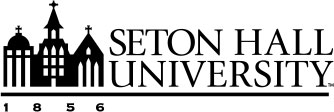James Davidson, Ph.D
Lecturer
Department of Mathematics and Computer Science

(973) 761-5168
Email

McQuaid Hall
Room 211

## James Davidson, Ph.D

I am the course coordinator for MATH1205: Finite Math with Calculus for Business and MATH1015: Precalculus. I also teach the Analysis sequence (MATH 3515 and 4516) for juniors/seniors. I also have taught Calculus 1 (MATH1401), 2 (MATH1511), and 3 (MATH 2511); Probability and Statistics (MATH1203 and MATH2111), and Linear Algebra and Differential Equations (MATH 2810). I have represented the department at the annual Pirate Preview as well as volunteered at the Andrushkiw Mathematics Competition for High School students.'

I have an interest in Differential Equations, Real Analysis, and Complex Analysis.